# Smart Energy and Utility Monitoring System

Project SEUMS - A smart energy monitoring system that will ease the inspection, monitoring, analysis, and calculation of energy fare.

IntermediateFull instructions provided20 hours624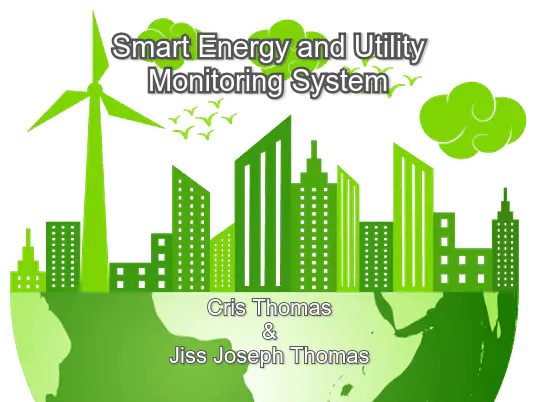## Things used in this project

### Hardware components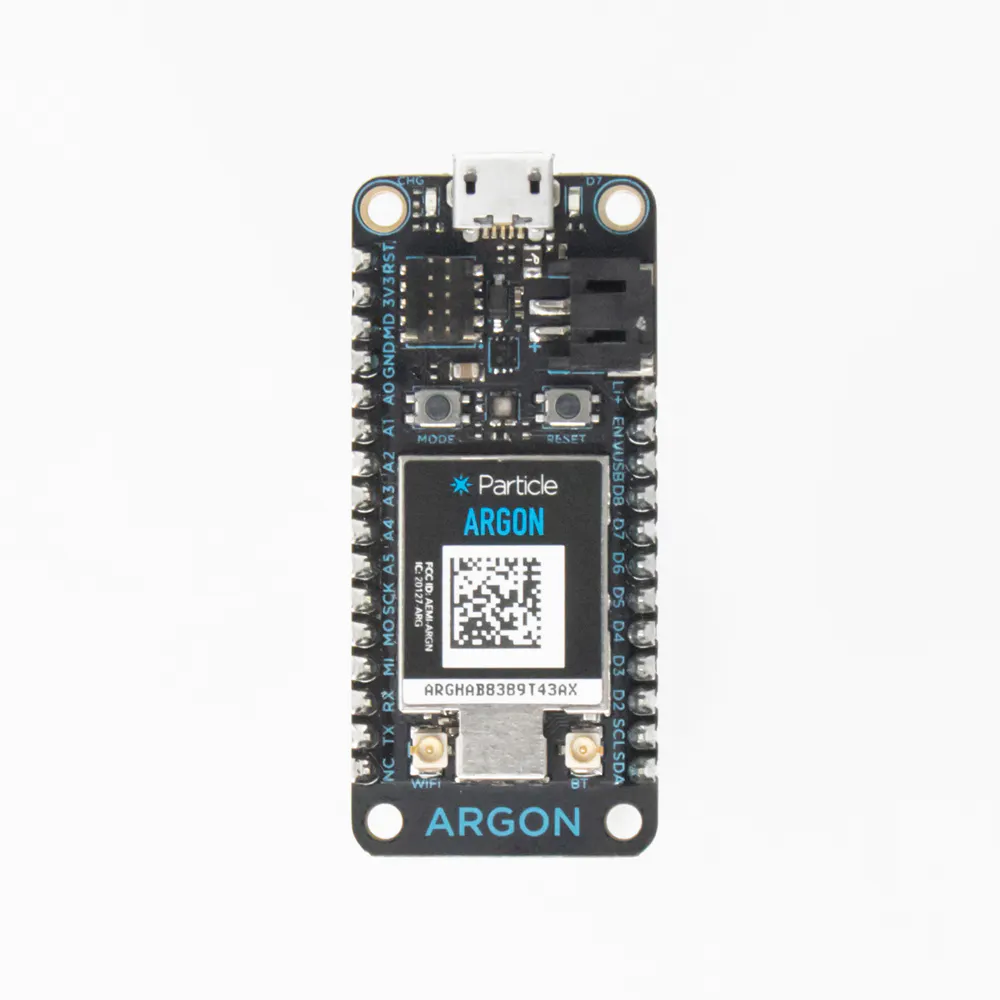Particle Argon
×1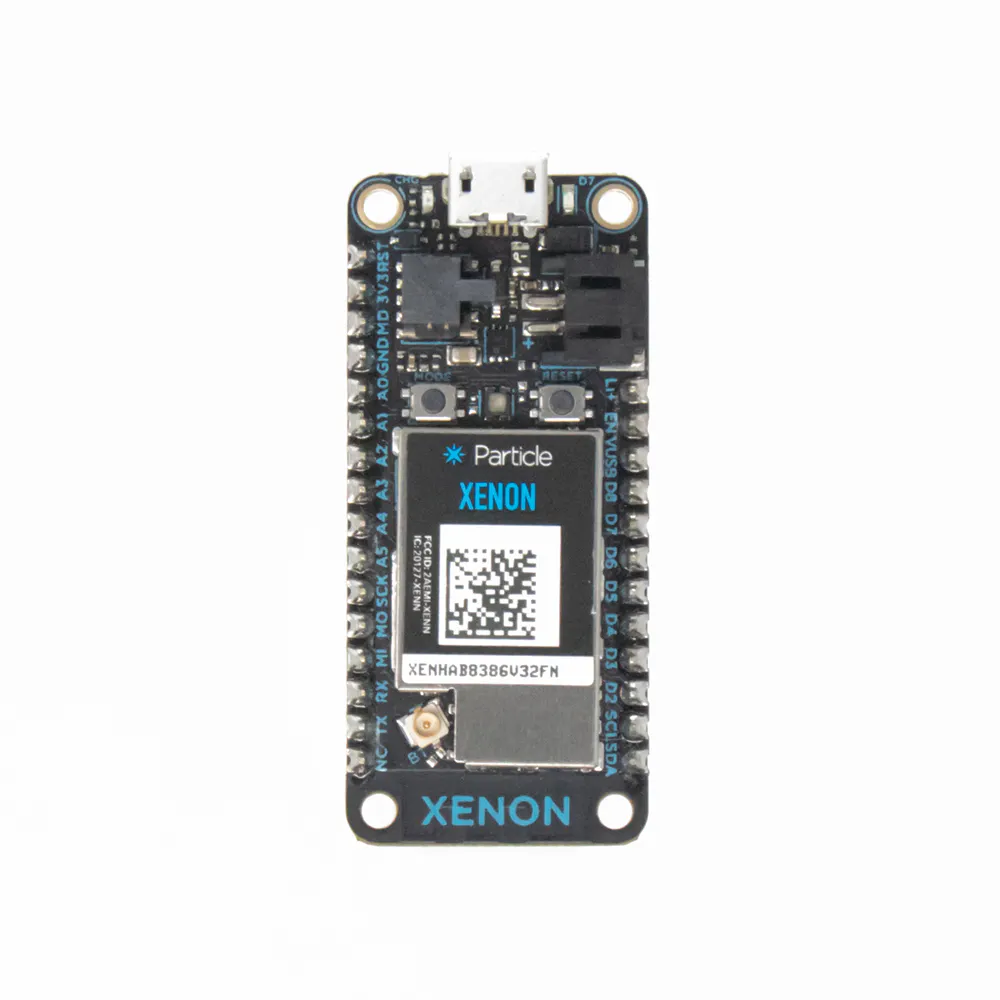Particle Xenon
×1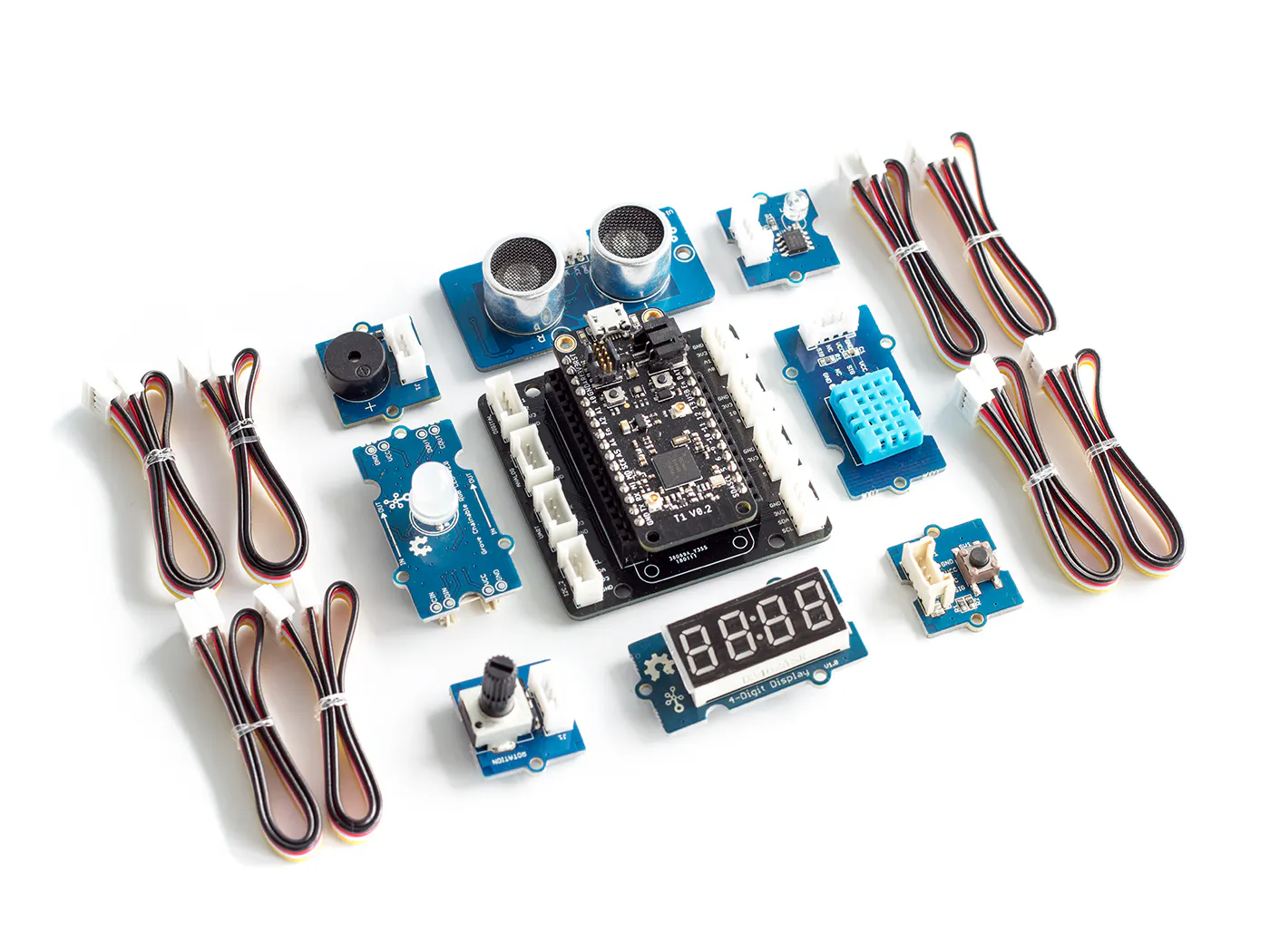Seeed Grove Starter Kit for Particle Mesh
×1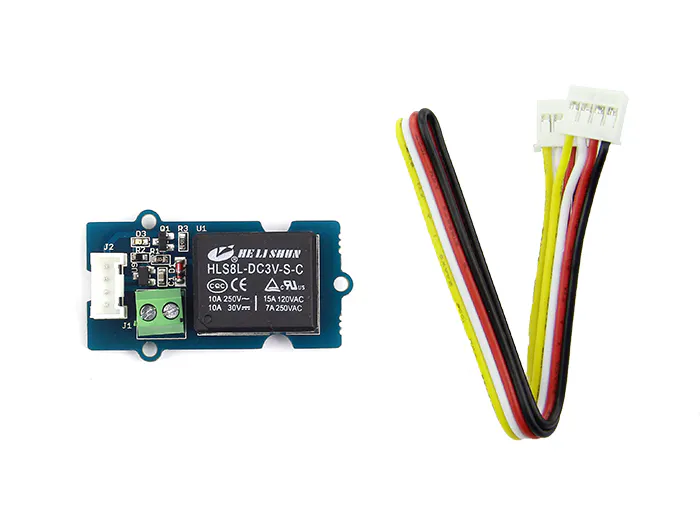Seeed Grove - Relay
×1
 Seeed Grove 5v Current Sensor
×1
 Seeed Grove 4 digit display
×1

### Software apps and online servicesParticle Build Web IDE

## Schematics

### System Diagram

All connections are standard Grove Connections as per connections described in the project page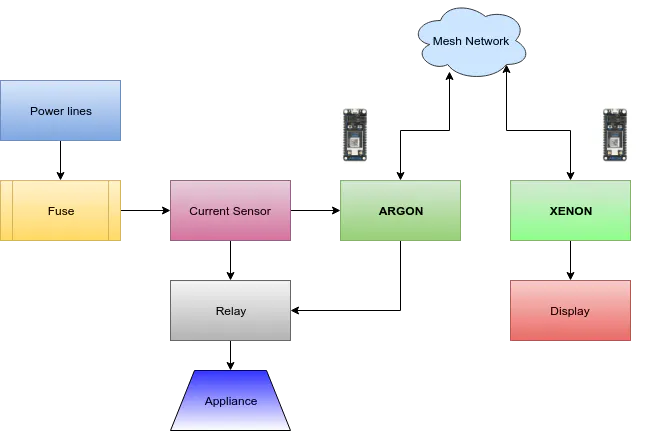## Code

### SEUMS.ino

Arduino
Smart Energy and Utility Monitoring System
```/*
* Project SEUMS
* Description: Smart Energy and Utility Monitoring System
* Author: Cris Thomas, Jiss Joseph Thomas
* Date: June 24, 2019
*/

#include "TM1637.h"

#define CLK D2
#define DIO D3

TM1637 tm1637(CLK,DIO);

void dispNum(unsigned int num);
void send_online();
void get_Wh();
void check_usage();

long int TimeRef = 0;
long int TimeNow = 0;
bool send_data = false;

const int relay_pin = D4;
const int current_pin = A0;
const int house_id = 1;
const int averageValue = 10;
const double Wh_threshold = 900;

int sensorValue = 0;
int RefVal = 20;
float sensitivity = 1000.0 / 800.0;
float Vref = 265;
float Vpp = 0;                          // peak-peak voltage
float Vrms = 0;                         // rms voltage
float Irms = 0;                         // rms current
float Supply_Voltage = 233.0;           // reading from DMM
float Vcc = 5.0;                        // ADC reference voltage
float power = 0;                        // power in watt
float Wh =0 ;                           // Energy in kWh

unsigned long last_time =0;
unsigned long current_time =0;
unsigned long interval = 100;
unsigned int Sensitivity = 185;
unsigned int calibration = 100;
unsigned int pF = 85;
unsigned int energyTariff = 8.0;        // Energy cost in EUR per unit (kWh)

float bill_amount = 0;                  // 30 day cost as present energy usage incl approx PF

void setup()
{
TimeRef = millis();
tm1637.set();
tm1637.init();
tm1637.set(BRIGHT_TYPICAL);
tm1637.point(POINT_ON);
}
void loop()
{
TimeNow = millis();
if (TimeNow > TimeRef + 500)        //publish data every 50 millisecond
{
TimeRef = millis();
send_online();
}

get_Wh();
check_usage();

Particle.variable("Wh", &Wh, DOUBLE);
Particle.variable("Bill_amount", &bill_amount, DOUBLE);
Particle.function("Relay_power",ToggleRelay);

dispNum(Wh);
delay(200);
}

void dispNum(unsigned int num)
{
int8_t TimeDisp[] = {0x01,0x02,0x03,0x04};

if(num > 9999) num = 9999;
TimeDisp = num / 1000;
TimeDisp = num % 1000 / 100;
TimeDisp = num % 100 / 10;
TimeDisp = num % 10;
tm1637.display(TimeDisp);
}

void send_online()
{
Particle.publish("House ID", String(house_id));
Particle.publish("Usage (Wh)", String(Wh));
Particle.publish("Amount", String(bill_amount));
String out = String::format("{\"Wh\":%.2f,\"BillAmount\":%.2f,}",Wh,bill_amount);
Particle.publish("JSON", out , PRIVATE, WITH_ACK);

}

void get_Wh()
{
for (int i = 0; i < averageValue; i++)
{
delay(2);
}

sensorValue = sensorValue / averageValue;
float unitValue= RefVal / 1024.0*1000 ;
float voltage = unitValue * sensorValue-Vref;
float current = (voltage - Vref) * sensitivity;

Vpp = voltage;
Vrms = (Vpp/2.0) *0.707;
Vrms = Vrms - (calibration / 10000.0);
Irms = (Vrms * 1000)/Sensitivity ;
if((Irms > -0.015) && (Irms < 0.008))
{
Irms = 0.0;
}
power= (Supply_Voltage * Irms) * (pF / 100.0);
last_time = current_time;
current_time = millis();
Wh = Wh+  power *(( current_time -last_time) /3600000.0) ;
bill_amount = Wh * (energyTariff/1000);
}

void check_usage()
{
if(Wh > Wh_threshold)
{
digitalWrite(relay_pin,LOW);
}
}

int ToggleRelay(String command)
{
if (command=="on") {
digitalWrite(relay_pin,HIGH);
return 1;
}
else if (command=="off") {
digitalWrite(relay_pin,LOW);
return 0;
}
else {
return -1;
}
}

```

## Credits

### Cris Thomas

13 projects • 49 followers
Electronics and Aerospace engineer with a dedicated history in Research and Development

### Jiss Joseph Thomas

10 projects • 39 followers
Co-Researcher Trainee (Artificial Intelligence) at Creopedia Business Intelligence Pvt.Ltd, Kochi,